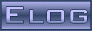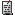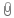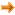Back Midas Rome Roody Rootana
 Midas DAQ System Not logged in12 May 2020, Stefan Ritt, Info, New ODB++ API20 May 2020, Konstantin Olchanski, Info, New ODB++ API20 May 2020, Stefan Ritt, Info, New ODB++ API20 May 2020, Pintaudi Giorgio, Info, New ODB++ API20 May 2020, Konstantin Olchanski, Info, New ODB++ API
Message ID: 1906     Entry time: 12 May 2020     Reply to this: 1913   1914   1915
 Author: Stefan Ritt Topic: Info Subject: New ODB++ API
```Since the beginning of the lockdown I have been working hard on a new object-oriented interface to the online database ODB. I have the code now in an initial state where it is ready for
testing and commenting. The basic idea is that there is an object midas::odb, which represents a value or a sub-tree in the ODB. Reading, writing and watching is done through this
object. To get started, the new API has to be included with

#include <odbxx.hxx>

To create ODB values under a certain sub-directory, you can either create one key at a time like:

midas::odb o;
o.connect("/Test/Settings", true);   // this creates /Test/Settings
o.set_auto_create(true);            // this turns on auto-creation
o["Int32 Key"] = 1;                 // create all these keys with different types
o["Double Key"] = 1.23;
o["String Key"] = "Hello";

or you can create a whole sub-tree at once like:

midas::odb o = {
{"Int32 Key", 1},
{"Double Key", 1.23},
{"String Key", "Hello"},
{"Subdir", {
{"Another value", 1.2f}
}
};
o.connect("/Test/Settings");

To read and write to the ODB, just read and write to the odb object

int i = o["Int32 Key];
o["Int32 Key"] = 42;
std::cout << o << std::endl;

This works with basic types, strings, std::array and std::vector. Each read access to this object triggers an underlying read from the ODB, and each write access triggers a write to the
ODB. To watch a value for change in the odb (the old db_watch() function), you can use now c++ lambdas like:

o.watch([](midas::odb &o) {
std::cout << "Value of key \"" + o.get_full_path() + "\" changed to " << o << std::endl;
});

Attached is a full running example, which is now also part of the midas repository. I have tested most things, but would not yet use it in a production environment. Not 100% sure if there
are any memory leaks. If someone could valgrind the test program, I would appreciate (currently does not work on my Mac).

Have fun!

Stefan

```
 Attachment 1: odbxx_test.cxx  4 kB  Uploaded 12 May 2020
```/********************************************************************\

Name:         odbxx_test.cxx
Created by:   Stefan Ritt

Contents:     Test and Demo of Object oriented interface to ODB

\********************************************************************/

#include <string>
#include <iostream>
#include <array>
#include <functional>

#include "odbxx.hxx"
#include "midas.h"

/*------------------------------------------------------------------*/

int main() {

cm_connect_experiment(NULL, NULL, "test", NULL);
midas::odb::set_debug(true);

// create ODB structure...
midas::odb o = {
{"Int32 Key", 42},
{"Bool Key", true},
{"Subdir", {
{"Int32 key", 123 },
{"Double Key", 1.2},
{"Subsub", {
{"Float key", 1.2f},     // floats must be explicitly specified
{"String Key", "Hello"},
}}
}},
{"Int Array", {1, 2, 3}},
{"Double Array", {1.2, 2.3, 3.4}},
{"String Array", {"Hello1", "Hello2", "Hello3"}},
{"Large Array", std::array<int, 10>{} },   // array with explicit size
{"Large String", std::string(63, '\0') },  // string with explicit size
};

// ...and push it to ODB. If keys are present in the
// ODB, their value is kept. If not, the default values
// from above are copied to the ODB
o.connect("/Test/Settings", true);

// alternatively, a structure can be created from an existing ODB subtree
midas::odb o2("/Test/Settings/Subdir");
std::cout << o2 << std::endl;

// retrieve, set, and change ODB value
int i = o["Int32 Key"];
o["Int32 Key"] = i+1;
o["Int32 Key"]++;
o["Int32 Key"] *= 1.3;
std::cout << "Should be 57: " << o["Int32 Key"] << std::endl;

// test with bool
o["Bool Key"] = !o["Bool Key"];

// test with std::string
std::string s = o["Subdir"]["Subsub"]["String Key"];
s += " world!";
o["Subdir"]["Subsub"]["String Key"] = s;

// test with a vector
std::vector<int> v = o["Int Array"];
v = 10;
o["Int Array"] = v;        // assign vector to ODB object
o["Int Array"] = 2;     // modify ODB object directly
i = o["Int Array"];     // read from ODB object
o["Int Array"].resize(5);  // resize array
o["Int Array"]++;          // increment all values of array

// test with a string vector
std::vector<std::string> sv;
sv = o["String Array"];
sv = "New String";
o["String Array"] = sv;
o["String Array"] = "Another String";

// iterate over array
int sum = 0;
for (int e : o["Int Array"])
sum += e;
std::cout << "Sum should be 11: " << sum << std::endl;

// creat key from other key
midas::odb oi(o["Int32 Key"]);
oi = 123;

// test auto refresh
std::cout << oi << std::endl;    // each read access reads value from ODB
oi.set_auto_refresh_read(false); // turn off auto refresh
std::cout << oi << std::endl;    // this does not read value from ODB
std::cout << oi << std::endl;

midas::odb ox("/Test/Settings/OTF");
ox.delete_key();

// create ODB entries on-the-fly
midas::odb ot;
ot.connect("/Test/Settings/OTF", true);   // this forces /Test/OTF to be created if not already there
ot.set_auto_create(true);        // this turns on auto-creation
ot["Int32 Key"] = 1;             // create all these keys with different types
ot["Double Key"] = 1.23;
ot["String Key"] = "Hello";
ot["Int Array"] = std::array<int, 10>{};
ot["Subdir"]["Int32 Key"] = 42;
ot["String Array"] = std::vector<std::string>{"S1", "S2", "S3"};
std::cout << ot << std::endl;

o.read();                        // re-read the underlying ODB tree which got changed by above OTF code
std::cout << o.print() << std::endl;

// iterate over sub-keys
for (auto& oit : o)
std::cout << oit.get_odb()->get_name() << std::endl;

// print whole sub-tree
std::cout << o.print() << std::endl;

// dump whole subtree
std::cout << o.dump() << std::endl;

// delete test key from ODB
o.delete_key();

// watch ODB key for any change with lambda function
midas::odb ow("/Experiment");
ow.watch([](midas::odb &o) {
std::cout << "Value of key \"" + o.get_full_path() + "\" changed to " << o << std::endl;
});

do {
int status = cm_yield(100);
if (status == SS_ABORT || status == RPC_SHUTDOWN)
break;
} while (!ss_kbhit());

cm_disconnect_experiment();
return 1;
}```
ELOG V3.1.4-2e1708b5